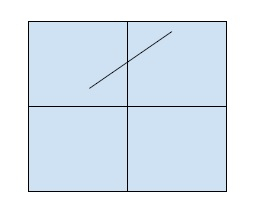# Program to find slope of a line in C++

In this problem, we are given the coordinates of two points of a line. Our task is to create a Program to find slope of a line in C++.

Problem Description − We will find the slope of the line using the coordinates of two points on the line that are given.

Let’s take an example to understand the problem

## Input

p1(-1, 1), p2(3, 3)## Output

½ = 0.5

## Solution approach

To find the slope of the line, we will use the geometrical formula defined to find the slope of a line using any two points P1(x1, y1) and P2(X2, Y2) that lie on the line.

Slope = (Y2 - Y1)/(X2 - X1)

Program to illustrate the working of our solution

## Example

Live Demo

#include<iostream>
using namespace std;
float calcSlope(float point){
float slope = ( (point-point) / (point - point));
return slope;
}
int main() {
float points = {{-1, 1}, {3, 3}};
cout<<"The slope of the line is "<<calcSlope(points);
}

## Output

The slope of the line is 0.5